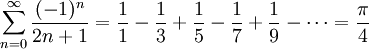Name: __________________________ Student ID: __________________________
1. Name the five steps to top-down
problem solving as described in the text.
(It's okay if you can't remember the exact wording; the concepts are more important.) 

 ___________ ___________ ___________ ___________ ___________
2. Name the five computer program flow abstractions described in the text. 

 ___________ ___________ ___________ ___________ ___________
3. Write "yes" or "no" next to each of the following six strings to indicate if it is a legal Python identifier. 
 numApples ______ 21st_birthday ______ prime-factor ______ for ______ _illegal ______ L337_hAX0rz ______
4. Evaluate each of the following Python expressions, or if it gives an error, indicate why. 
 (5 % 12 == 2) or False and not False _________________________ 2 ** 3+16.2 // 5 _________________________ 0!=-0 and 0-0/0<0 _________________________ 'pi' + 2*'z' + 'a' _________________________ 2*range(3) _________________________
5. The following loop was intended to count down from ten to one, then print "Blast off!". What is wrong with the loop? How would you fix it? 
```counter = 10.0
while counter != 1.0:
print int(counter),
counter -= 1.0
print "Blast off!"

```
6. Recall that in Python int/float/bool parameters are essentially passed by value, and lists/dictionary parameters are essentially passed by reference. What is printed by the following block of Python code? 
```def double_me(x):
x *= 2
y = 3.0
z = range(3)
double_me(y)
double_me(z)
print 'y =', y, ', z =', z
```
7. Leibniz' formula for calculating pi is an infinite series:In the space below the next problem, write pseudocode for a function procedure leibniz() that returns an estimate of pi. A parameter should be used to tell the function the maximum value of n to use in the sum. For example, leibniz(3) should add terms up to and including n==3. 

8. On a separate sheet of paper, write a complete Python program, declaring the procedure leibniz(). The body of the program should invoke leibniz() a few times to test it out, using a different number of terms of the infinite series. (You could also let the user interactively specify the parameter to leibniz().) 

Docstrings (including preconditions) are required for your whole module and for each function. You are not required to comment your code on this exam, however if your code is incorrect, your comments may earn you partial credit if they show good design thinking. Little partial credit will be given for uncommented incorrect code.